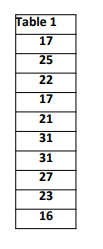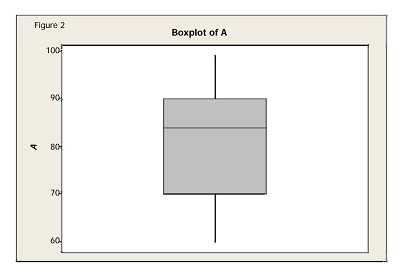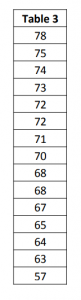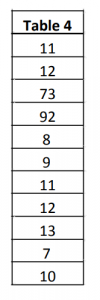## Lean Six Sigma Green Belt Sample Paper: Background

1. Please carry out Anderson‐ Darling normality test for the data provided in Table 1. What is the p‐value and inference at 5% significance level?2. What percentage of points fall between 70 and 90 in the box plot shown in figure?3. For the data provided in table 3, please compute Arithmetic Mean, sample standard deviation and median4. For the data provided in table 4, which of the following measures is a robust measure of central tendency?5. Assuming the data follows perfect normal distribution, what percentage of the values fall within ± 2 sigma of the mean?
6. If Sample Variance is 24.01, Range is 10, Sample standard deviation is equal to
7. What is sometimes called the 8th waste in Lean?
8. A box plot displays:
9. Which of the following is a discrete distribution?
10. Radical Re‐engineering is commonly associated with
11. In a normal standard distribution,
12. According to John Kotter, what is the most common reason for project failure?
13. Which graphical tool plots quartiles?
14. A good Six Sigma DMAIC project is one, for which